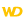HelpWLanguageWLanguage functionsStandard functionsNumeric values management functionsFinancial functionsFinRepayVal (Example)Amount of each payment for an investmentFinCurrentVal (Example)FinCurrentValFinDecreasingRedemption (Example)FinDecreasingRedemptionFinError (Example)FinErrorFinFutureVal (Example)FinFutureValFinInterestRate (Example)FinInterestRateFinLinearRedemption (Example)FinLinearRedemptionFinNetCurrentVal (Example)FinNetCurrentValFinPaymentNb (Example)FinPaymentNbFinPeriodInterest (Example)FinPeriodInterestFinRedemption (Example)FinRedemptionFinRepayVal (Example)FinRepayValFinancial constantsFinancial functions
 Amount of each payment for an investment FinRepayVal (Example)See the associated help page Amount of each payment for an investmentThe following code is used to calculate the amount of each payment for an investment. The initial capital, the total number of periods and the interest rate are entered by the user in edit controls (EDT_InitCapital, EDT_NbPeriods, EDT_Rate). // --Click code on BTN_RepaymentVal// Declare the variableResRepaymentVal is real // Calculate the current valueResRepaymentVal = FinRepayVal(-EDT_InitCapital, EDT_NbPeriods, EDT_Rate)IF FinError() = 0 THEN Info("The amount of each payment is: " + ResRepaymentVal)ELSE // Display the error message Error(ErrorInfo(errMessage))END Minimum version required Version 9 This page is also available for…